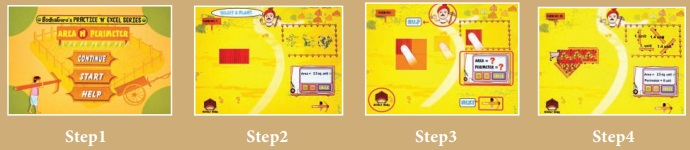Home | | Science 6th Std | Some open ended questions

# Some open ended questions

The school authority planned to conduct a mini marathon race within the school campus. They decided that the running distance to be 2 kilometres. Is it possible to have a school campus with circumference of 2km? Discuss with your friends of how big the campus should be?

Some open ended questions

* The school authority planned to conduct a mini marathon race within the school campus. They decided that the running distance to be 2 kilometres. Is it possible to have a school campus with circumference of 2km? Discuss with your friends of how big the campus should be?

Given: school campus circumference = 2km

Yes, it is possible to have a school campus with circumference of 2km.

Whatever may be shape of the school if the perimeter of the school is more than or equal to 2km a mini marathon race within the school campus is possible.

* Give other options if it is not a big campus.

If the perimeter of the school is not more than or equal to 2km running more than one time (i.e., 2, 3 .... Rounds) and make the distance equal to 2km a mini marathon race within the school campus is possible.

* Is the distance in the sea also calculated in kilometres? How is it possible to calculate the distance in sea water? Explore!

Yes, the distance in the sea is also calculated in kilometers.

It is possible to calculate the distance in sea water by special methods like radar method, echo method.

* We know that the distance between celestial bodies is calculated in terms of light year. (Ah! Unit  of distance in terms of year???) Yes, it’s the distance travelled by light in one year. Now without calculator find how many kilometres light would have travelled in a year. (No Calculator). Get the speed of light from your class teacher.

Light travels 3 × 108 m in one second or 3 lakhs kilometer in one second. In one year we have 365 days. The total number of seconds in one year is equal to 365 × 24 × 60 × 60 = 3.153 × 107 second.

1 light year = (3.153 × 107) × (3 × 108) = 9.46 × l015m.

* We see that the distances between Chennai and Madurai is ‘462’ kms. But from which point to which point is this distance calculated?. As we are science students we need to know it with the precision. Is it between the two bus stands? Or between the two railway stations? Discuss and figure it out. Check your answers with your class teacher.

If we travelling through the road it is the distance between two bus stand. If we travelling through the train it is the distance between two railway stations.

* A person needs to drink two litres of water a day. Note down how much water you drink each day? Make a rough calculation and check if you are drinking the required amount of water.

Morning - 200 ml

After brakfast - 500 ml

At 11 am (app) - 200 ml

After lunch - 500 ml

At 3 pm (app) - 200 ml

After dinner - 500 ml

Total - 2100 ml = 2.1 Litre

ICT CORNER

AREA & PERIMETER

Lets play withArea N PerimeterSteps:

* Access the application by typing Area N Perimeter or install with the help of the link given below or the given QR code

* Open the Application and click START button.

* You can see the field whose area is to be measured. Drag and put the tiles on field.

* Use the (+) and (-) to find out the area of the given field.

* You can view your whole results by clicking the RESULT button.URL:

AreaNPerimeter

Tags : Measurements | Term 1 Unit 1 | 6th Science , 6th Science : Term 1 Unit 1 : Measurements
Study Material, Lecturing Notes, Assignment, Reference, Wiki description explanation, brief detail
6th Science : Term 1 Unit 1 : Measurements : Some open ended questions | Measurements | Term 1 Unit 1 | 6th Science